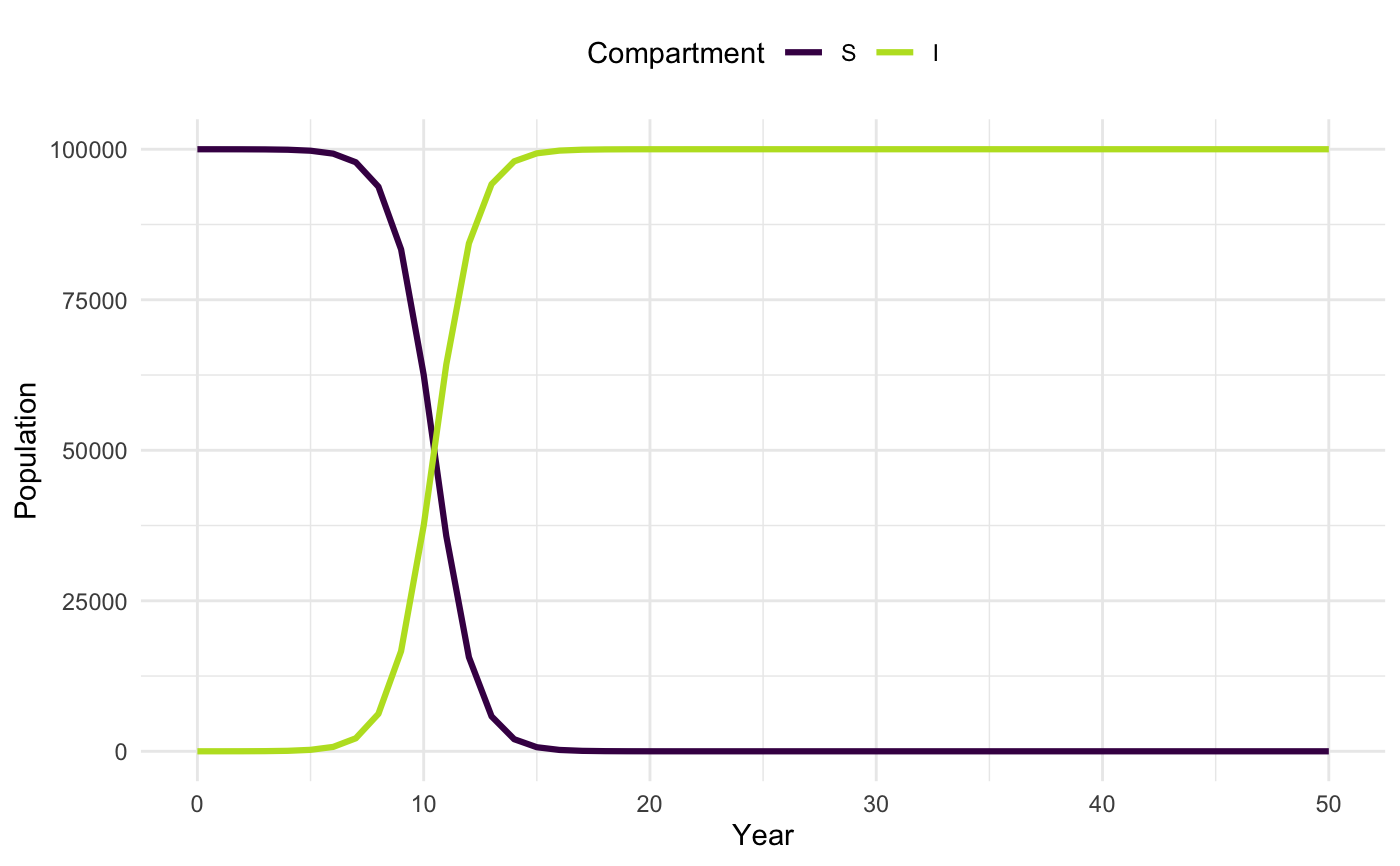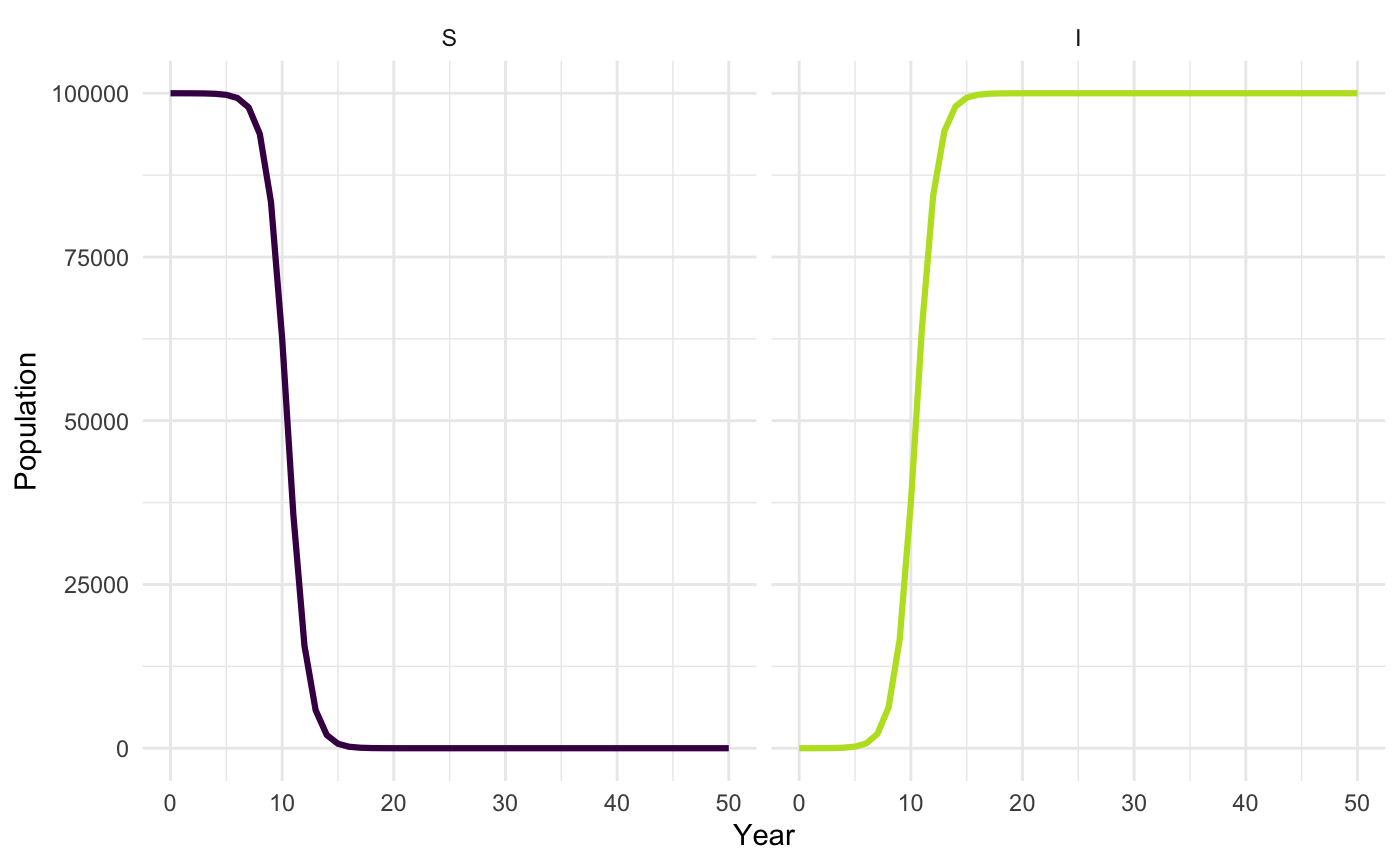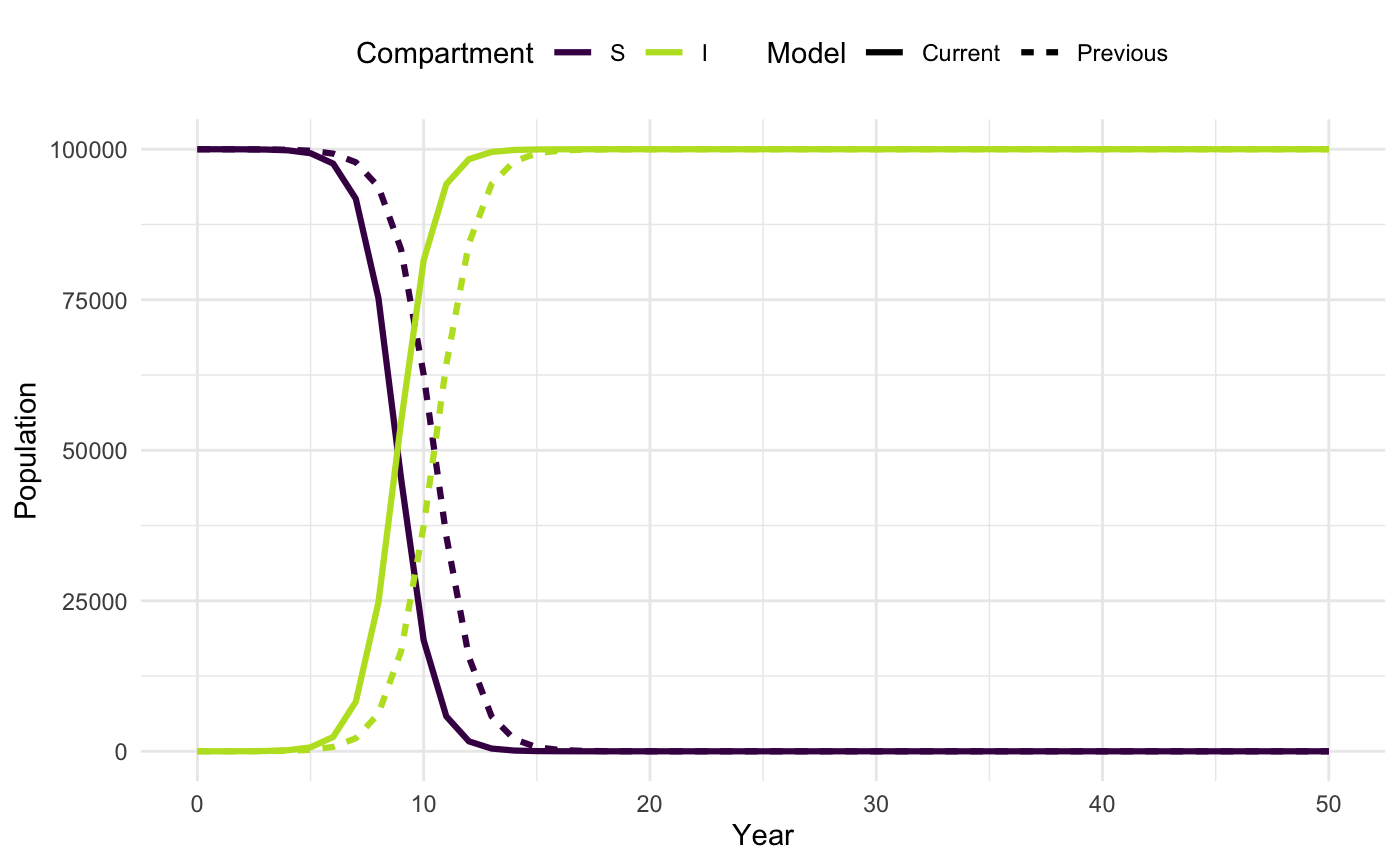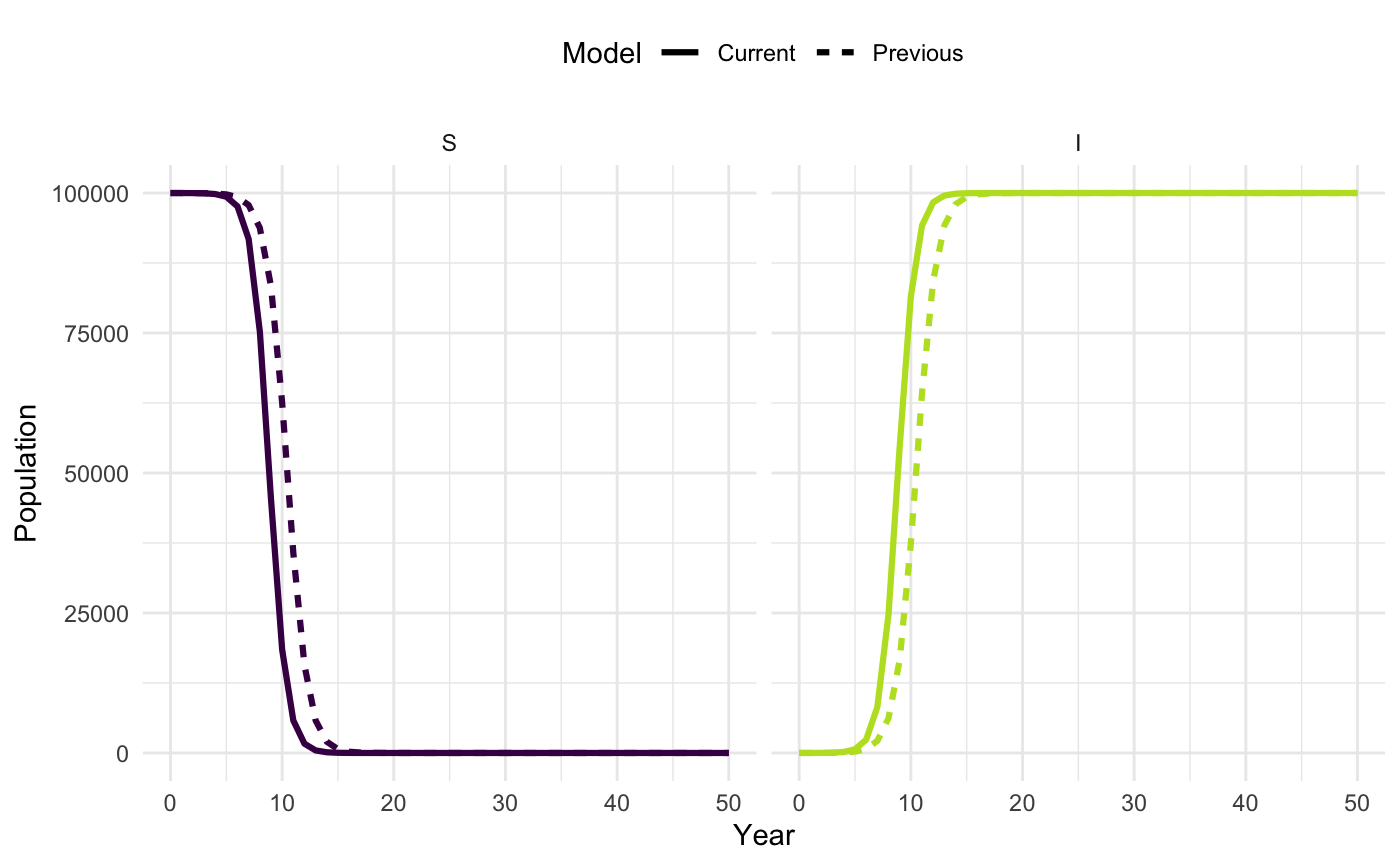Make separate plots for each model compartment. Assumes model output is structured as that produced from solve_ode.

plot_model(sim, prev_sim = NULL, model_labels = NULL, facet = TRUE)

## Arguments

sim A tibble of model output as formatted by solve_ode. Optionally a list of simulations can be passed when comparing multiple model runs. A second tibble of model output formatted as for sim. Used to compare to model runs. Can only be supplied if sim is not a list. A character vector of model names. Defaults to c("Current", "Previous") when two model simulations are used and the list names when sim is a list. If sim is unnamed the index of the list is used. Logical, defaults to TRUE. If FALSE then the plot will not be faceted otherwise it will be.

## Value

A Plot of each model compartments population over time.

## Examples


## Intialise
N = 100000
I_0 = 1
S_0 = N - I_0
R_0 = 1.1
beta = R_0

##Time for model to run over
tbegin = 0
tend = 50
times <- seq(tbegin, tend, 1)

##Vectorise input
parameters <- as.matrix(c(beta = beta))
inits <- as.matrix(c(S = S_0, I = I_0))

sim <- solve_ode(model = SI_ode, inits, parameters, times, as.data.frame = TRUE)

plot_model(sim, facet = FALSE)plot_model(sim, facet = TRUE)## Compare with an updated model run

#'## Intialise
R_0 = 1.3
beta = R_0
parameters <- as.matrix(c(beta = beta))

new_sim <- solve_ode(model = SI_ode, inits, parameters, times, as.data.frame = TRUE)

plot_model(new_sim,sim, facet = FALSE)plot_model(new_sim, sim, facet = TRUE)## Passing in the simulations as a list
plot_model(list("Current" = new_sim, "Previous" = sim), facet = TRUE)